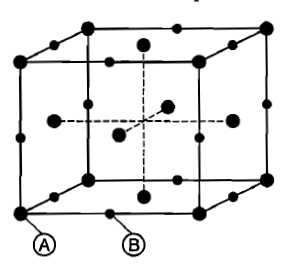The elements of symmetry in a crystal are:-
(a) plane of symmetry
(b) axis of symmetry
(c) centre of symmetry
(d) all of these

Concept Questions :-

Introduction and Type of Crystal System
High Yielding Test Series + Question Bank - NEET 2020

Difficulty Level:

Compute the percentage void space per unit volume of unit cell in zinc fluoride structure.

1. 15.03%

2. 22.18%

3. 18.23%

4. 25.07%

Concept Questions :-

High Yielding Test Series + Question Bank - NEET 2020

Difficulty Level:

Which pairs shows isomorphism?
(a) KNO3, NaNO3
(b) Cr2O3, FeO
(c) Both (a) and (b)
(d) Ferrimagnetic

Concept Questions :-

Introduction and Type of Crystal System
High Yielding Test Series + Question Bank - NEET 2020

Difficulty Level:

For a solid with the following structure, the co-ordination number of the point B is:(a) 3

(b) 4

(c) 5

(d)6

Concept Questions :-

High Yielding Test Series + Question Bank - NEET 2020

Difficulty Level:

TiO2 is well known example of:

(a) triclinic system

(b) tetragonal system

(c) monoclinic system

(d) none of these

Concept Questions :-

Introduction and Type of Crystal System
High Yielding Test Series + Question Bank - NEET 2020

Difficulty Level:

How many octahedral and tetrahedral holes are present per unit cell in a face centred cubic arrangement of atoms?

(A) 8,4

(B) 1.2

(C) 4.8

(D) 2.1

Concept Questions :-

Introduction and Type of Crystal System
High Yielding Test Series + Question Bank - NEET 2020

Difficulty Level:

The density of KCl is 1.9893 g cm-3 and the length of a side unit cell is 6.29082 Å as determined by X-rays diffraction. The value of Avogadro’s number calculated from these data is:-

1. 6.017 x 1023

2. 6.023 x 1023

3. 6.03 x 1023

4. 6.017 x 1019

Concept Questions :-

Density/Formula/packing fraction/ Semiconductors
High Yielding Test Series + Question Bank - NEET 2020

Difficulty Level:

Frenkel defect is noticed in:

(a) AgBr

(b) ZnS

(c) Agl

(d) all of these

Concept Questions :-

Imperfections in Solids
High Yielding Test Series + Question Bank - NEET 2020

Difficulty Level:

Close packing is maximum in the crystal lattice of:

(a) simple cubic
(b) face centred
(c) body centred
(d) none of these

Concept Questions :-

Density/Formula/packing fraction/ Semiconductors
High Yielding Test Series + Question Bank - NEET 2020

Difficulty Level:

A spinel is an important class of oxides consisting of two types of metal ions with the oxide ions arranged in ccp layers. The normal spinel has 1/8th of the tetrahedral void occupied  by one type of metal and one half of the octahedral voids occupied by another type of metal ions. Such a spinel is formed by Zn2+, Al3+ and O2- with Zn2+ in tetrahedral void. Give the simplest formula of the spinel.

(A) ZnAl2O4

(B) ZnAl2O3

(C) ZnAlO

(D) None of these

Concept Questions :-

Magnetic Behaviour of an Element/Compound